# 人教版小学数学一年级上册第三单元同步练习及答案

1.3的前面是（ ），3的后面是（ ）。

2.5的前面是（ ），2的前面是（ ）。

3.按顺序写数。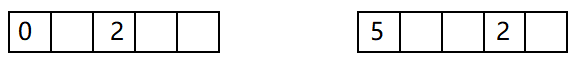4.在括号里填上正确的符号。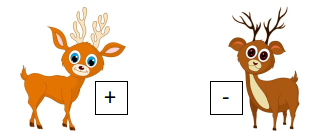4（ ）1=3 5（ ）3=2

2（ ）3=5 1（ ）2=3

2（ ）2=4 3（ ）1=2

5.在括号里填上正确的数。

2-（ ）=1 4+（ ）=5

2+（ ）=3 1+（ ）=4

5-（ ）=1 3-（ ）=0

6.在方框里面填上合适的数。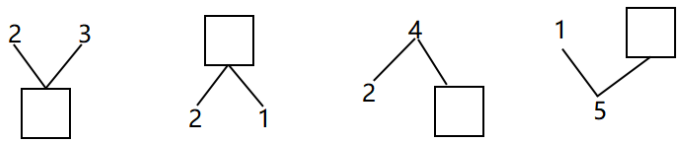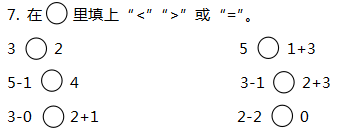8. 在（ ）填上合适的数。

1>（ ） 4<（ ）

5>（ ） 0=（ ）

3=（ ） （ ）>（ ）

1+3= 4-2=

3-3= 2+0=

1+2= 3+2=

1+4= 5-1=

5-2= 5-4=

4-0= 2+2=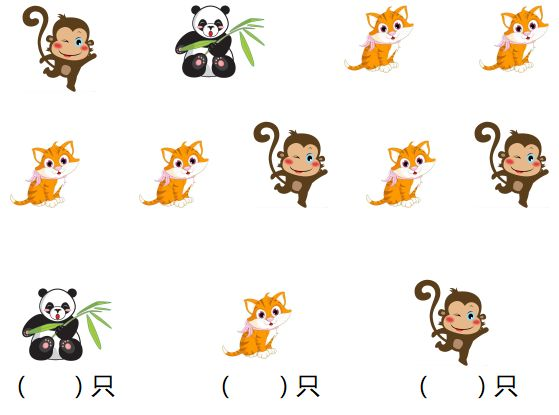（ ）>（ ）>（ ）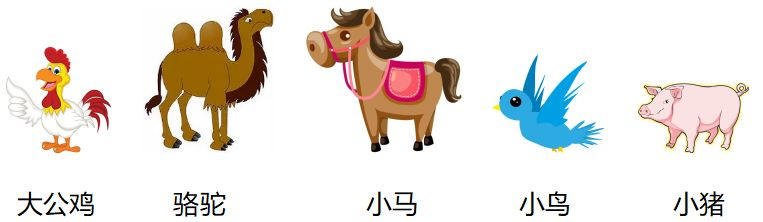1.图中一共有（ ）只动物。

2.小马的后面有（ ）只动物，从后面数小马排第（ ）。

3.从前面数，大公鸡排第（ ），小鸟排第（ ），小猪排第（ ）。

4.把中间的三只动物圈出来。

5.把从左边开始数是第二只的动物圈出来。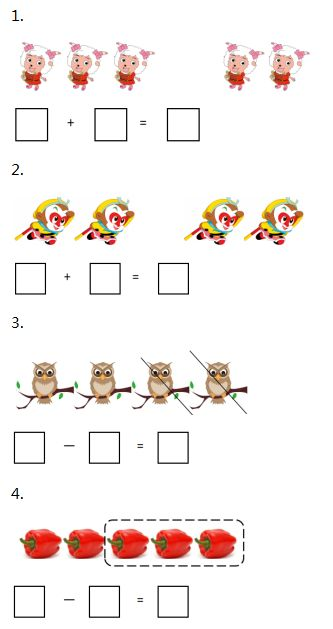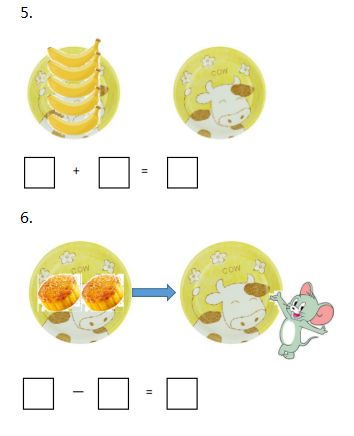1.（6分）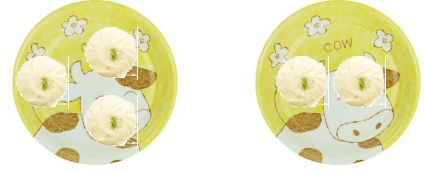2.（8分）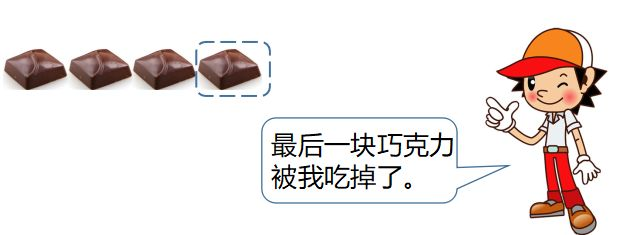1.2,4

2.4,1

3.如下图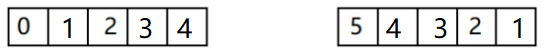4.

4（ - ）1=3 5（ - ）3=2

2（ + ）3=5 1（ + ）2=3

2（ + ）2=4 3（ - ）1=2

5.

2-（ 1 ）=1 4+（ 1 ）=5

2+（ 1 ）=3 1+（ 3 ）=4

5-（ 4 ）=1 3-（ 3 ）=0

6. 5,3,2,4

7.>,>,=,<,=,=

8.

1>（ 0 ）

4<（ 5 ）

5>（ 1 ）【填0、1、2、3、4都可】

0=（ 0 ）

3=（ 3 ）

（ 1 ）>（ 0 ）【答案不唯一，合理即可】

1+3=4 4-2=2

3-3=0 2+0=2

1+2=3 3+2=5

1+4=5 5-1=4

5-2=3 5-4=1

4-0=4 2+2=4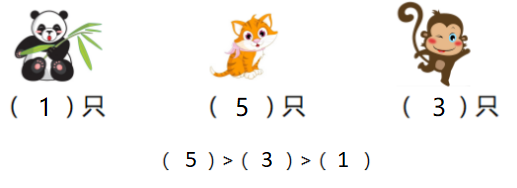1.5

2.2,3

3.1,4,5

4.如下图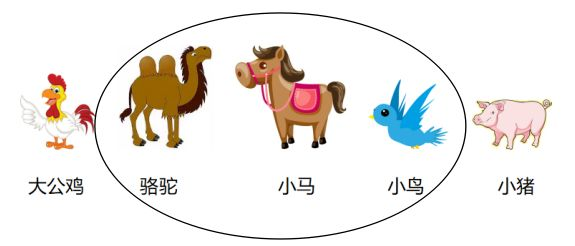5.如下图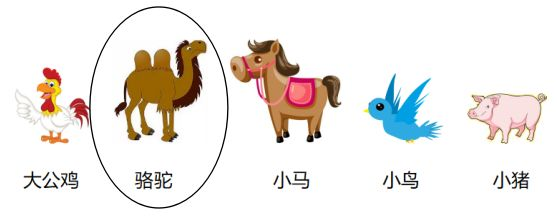1.3+2=5

2.2+2=4

3.4-2=2

4.5-3=2

5.5+0=5

6.2-2=0

1.3,2,5

2.4,1,3,4-1=3

###热门文章推荐95.6%的用户看完本文后，还关注了以下精彩公众号